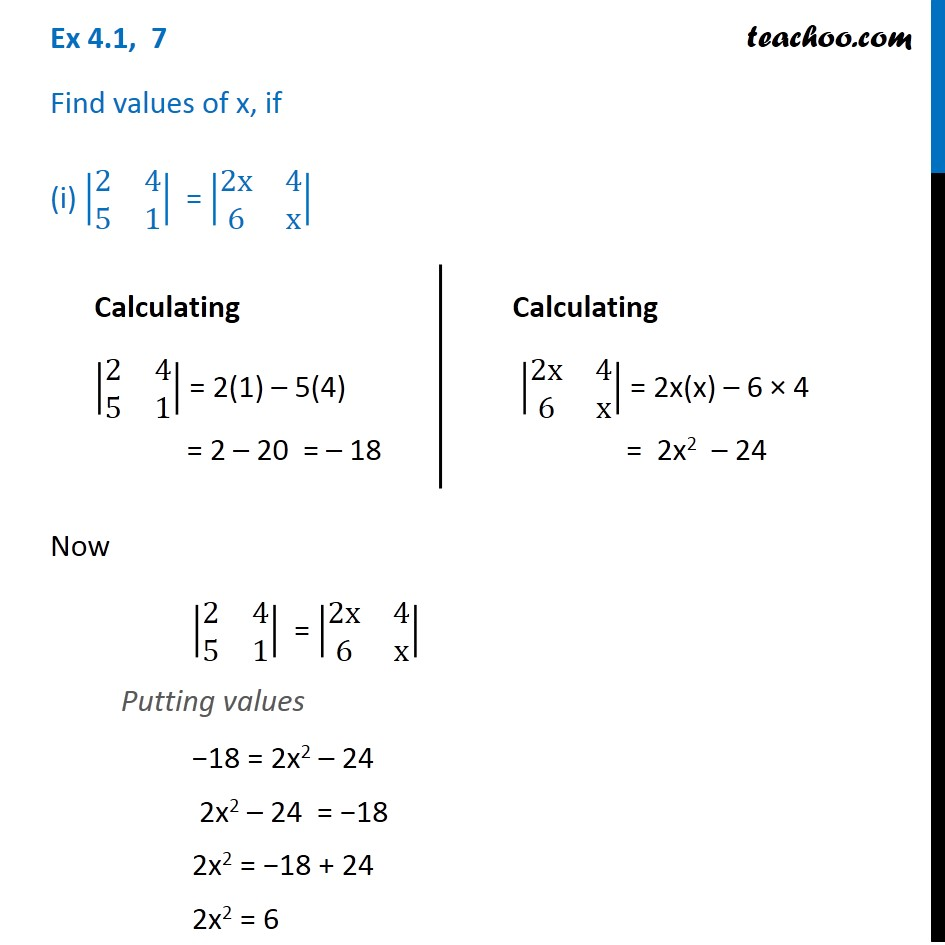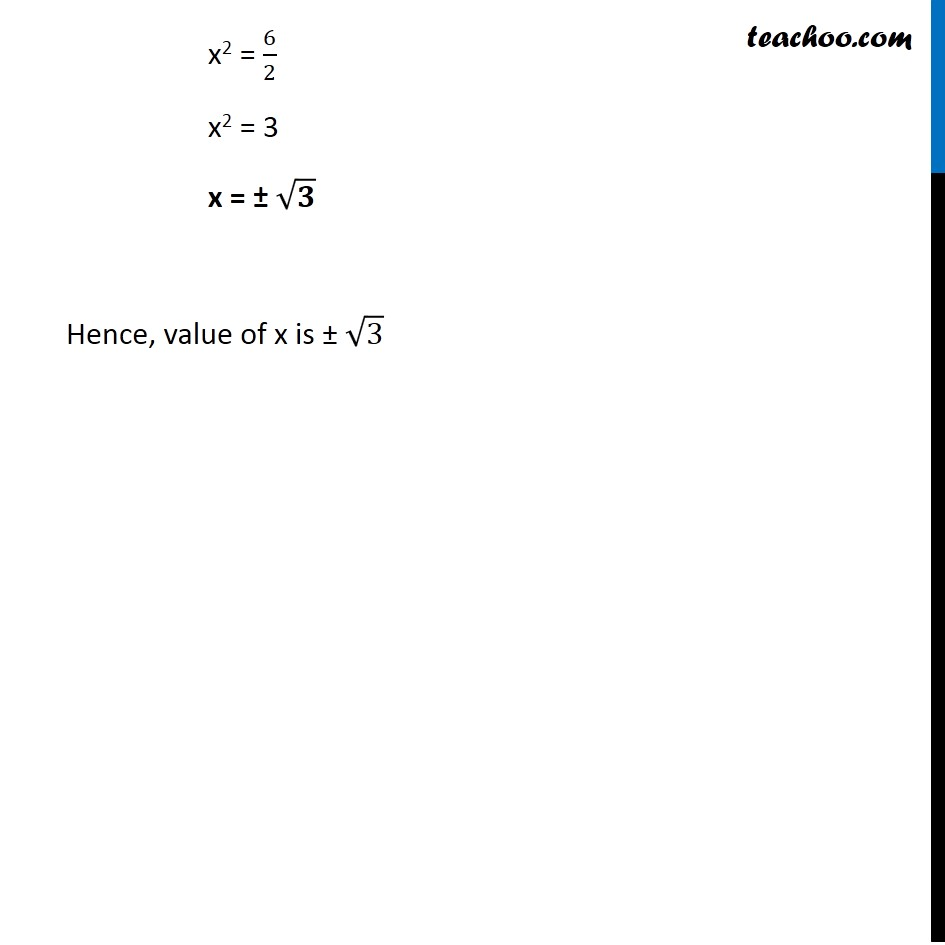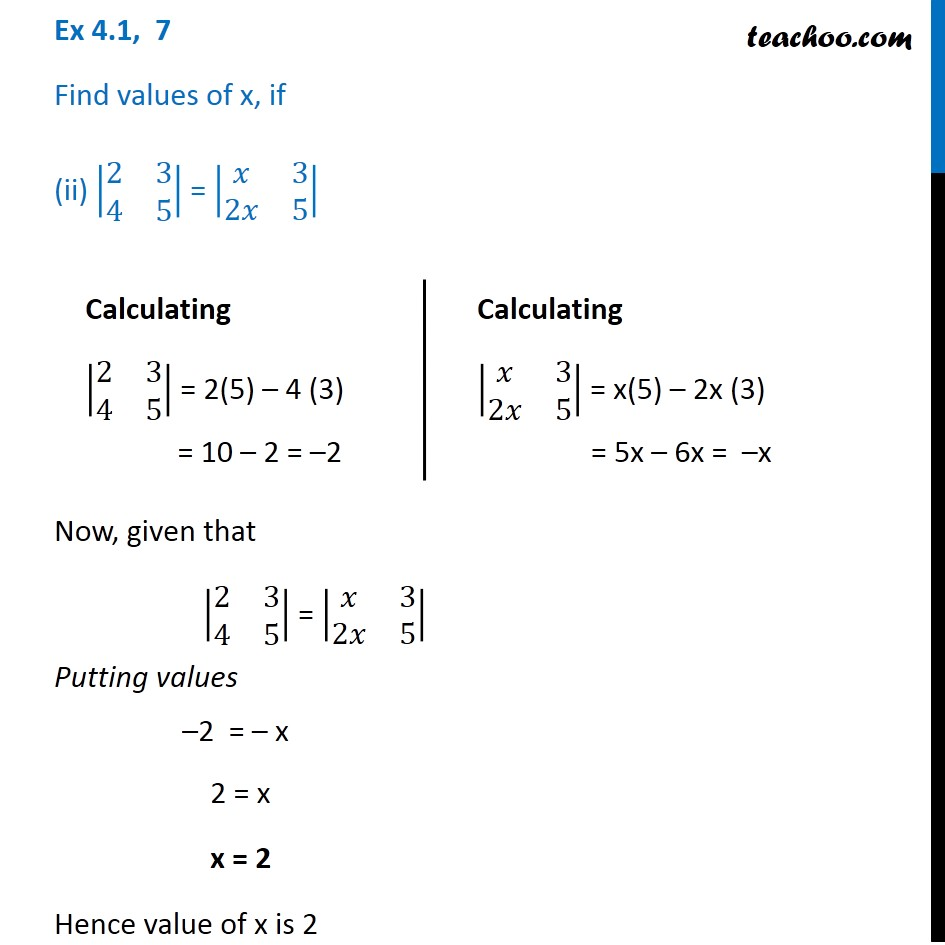Subscribe to our Youtube Channel - https://you.tube/teachoo

1. Chapter 4 Class 12 Determinants
2. Serial order wise
3. Ex 4.1

Transcript

Ex 4.1, 7 Find values of x, if (i) |■8(2&4@5&1)| = |■8(2x&4@6&x)|Calculating |■8(2&4@5&1)| = 2(1) – 5(4) = 2 – 20 = – 18 Calculating |■8(2x&4@6&x)| = 2x(x) – 6 × 4 = 2x2 – 24 Now |■8(2&4@5&1)| = |■8(2x&4@6&x)| Putting values −18 = 2x2 – 24 2x2 – 24 = −18 2x2 = −18 + 24 2x2 = 6 x2 = 6/2 x2 = 3 x = ± √𝟑 Hence, value of x is ± √3

Ex 4.1